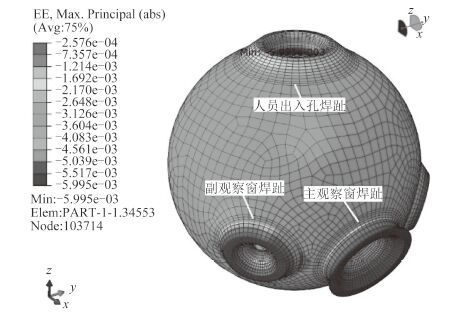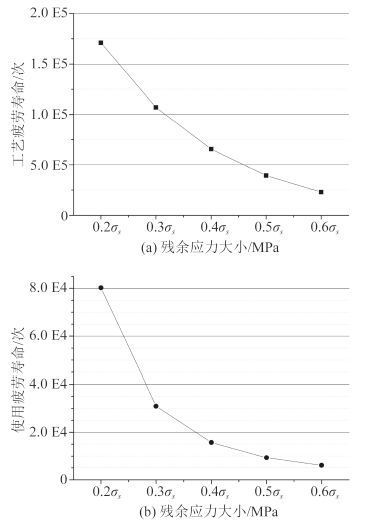﻿ 考虑残余应力效应的深潜器耐压壳体疲劳研究
 舰船科学技术2017, Vol. 39Issue (1): 32-36PDF

1. 上海交通大学 海洋工程国家重点实验室，上海 200240;
2. 高新船舶与深海开发装备协同创新中心，上海 200240;
3. 中国船级社规范与技术中心，上海 200135

Fatigue analysis of spherical shell considering residual stress
XUE Hong-xiang1,2, YANG Qi1,2, ZHENG Zu-zhong1,2, LUO Qiu-ming3, TANG Wen-yong1,2
1. State Key Laboratory of Ocean Engineering, Shanghai Jiaotong University, Shanghai 200240, China;
2. Collaborative Innovation Center for Advanced Ship and Deep-Sea Exploration, Shanghai 200240, China;
3. Rules & Technology Center, China Classification Society, Shanghai 200135, China
Abstract: In this paper, fatigue analysis is carried out considering welding residual stress for spherical shell of manned submersible based on local stress-strain method and unique curve model which could take negative stress ratio into account, and the influence of welding residual stress is discussed. Results show that fatigue life prediction result will be insecure if the influence of tensile residual stress was omitted. Tensile residual stress makes spherical shell under pressure suffer tensile-compressive cyclic load, thus must be taken into consideration when predicting fatigue life of spherical shell using crack propagation method. Fatigue life could be improved significantly by reducing tensile residual stress.
Key words: residual stress     spherical shell     fatigue life     local stress-strain method     unique curve model
0 引言

1 焊接残余应力分布及疲劳评估位置图 1 耐压球壳最大主应变分布 Fig. 1 Max principal strain fringe of spherical shell

 $\left\{ {\begin{array}{*{20}{l}} {{\sigma _R}(x) = {\sigma _R}(1- 4x/t),\left( {0 \leqslant x \leqslant t/2} \right),}\\ {{\sigma _R}(x) = {\sigma _R}(4x/t- 3),\left( {t/2 \leqslant x \leqslant t} \right)。} \end{array}{\rm{ }}} \right.$ (1)表 1 耐压球壳各疲劳评估位置最大主应变 Tab.1 Max principal strain value of different fatigue calculation positions
2 耐压球壳疲劳寿命预报 2.1 耐压球壳工艺疲劳寿命

 $\left\{ {\begin{array}{*{20}{l}} {\Delta \sigma \cdot \Delta \varepsilon = \frac{{{{\left( {{K_f}\Delta S} \right)}^2}}}{E},}\\ {\frac{{\Delta \varepsilon }}{2} = \frac{{\Delta {\varepsilon _e}}}{2} + \frac{{\Delta {\varepsilon _p}}}{2} = \frac{{\Delta \sigma }}{{2E}} + {{\left( {\frac{{\Delta \sigma }}{{2{K'}}}} \right)}^{\frac{1}{{{n'}}}}}。} \end{array}} \right.$ (2)

 $\frac{{\Delta \varepsilon }}{2} = \frac{{\Delta {\varepsilon _e}}}{2} + \frac{{\Delta {\varepsilon _p}}}{2} = \frac{{\sigma _f'- {\sigma _m}}}{E}{\left( {2{N_f}} \right)^b} + \varepsilon _f'{\left( {2{N_f}} \right)^c}。$ (3)表 2 钛合金材料参数 Tab.2 Material parameter of titanium alloy表 3 耐压球壳工艺疲劳寿命预报结果（单位：次） Tab.3 Prediction results of spherical shell crack initiation fatigue life（Unit：cycle）

2.2 耐压球壳使用疲劳寿命

 $\frac{{{\rm{d}}a}}{{{\rm{d}}N}} = C\left( {\Delta {K_E}^m- \Delta {K_{th}}^m} \right),$ (4)
 $\Delta {K_E} = M\Delta K,$ (5)
 $M = \left\{ {\begin{array}{*{20}{l}} {{{\left( {1- R} \right)}^{- {\beta _1}},}\;\;\;\;\;\;\;\;\;\;\;\;\;\;\;\;\;\;\;\;\;\;\;\left( {R < 0} \right),}\\ {{{\left( {1- R} \right)}^{- \beta },}\;\;\;\;\;\;\;\;\;\;\;\;\;\;\;\;\;\;\;\;\;\;\;\;\left( {0 \leqslant R < 0.5} \right),}\\ {{{(1.05- 1.4R + 0.6{R^2})}^{- \beta },}\;\;\;\;\;\left( {0.5 \leqslant R < 1} \right)。} \end{array}} \right.$ (6)表 4 耐压球壳使用疲劳寿命计算参数 Tab.4 Parameter for calculating crack propagation life

3 焊接残余应力大小的影响图 2 焊接残余应力大小的影响 Fig. 2 The influence of welding residual stress

4 结语

1）耐压球壳工艺疲劳寿命和使用疲劳寿命均大于球壳设计寿命，具有较高的安全裕度；

2）忽略开口焊趾处拉伸残余应力的影响将导致球壳工艺疲劳寿命预测结果偏于危险，并且实际疲劳破坏位置可能与预测结果出现偏差；

3）开口焊趾处拉伸残余应力使得整体受压的耐压球壳局部区域在下潜过程中承受拉-压循环载荷，降低耐压球壳的使用疲劳寿命，其影响不可忽略；

4）耐压球壳的工艺疲劳寿命和使用疲劳寿命均随焊接残余应力的提高而降低，降低拉伸焊接残余应力可以有效提高耐压球壳的疲劳寿命。

  ﻿李向阳, 崔维成, 张文明. 钛合金载人球壳的疲劳寿命可靠性分析[J]. 船舶力学 , 2006, 10 (2):82–86. LI Xiang-yang, CUI Wei-cheng, ZHANG Wen-ming. Fatigue life reliability analysis of Titanium manned spherical shell[J]. Journal of Ship Mechanics , 2006, 10 (2) :82–86.  李良碧, 罗广恩, 王自力. 大深度载人潜水器疲劳寿命有限元分析[J]. 江苏科技大学学报（自然科学版） , 2006, 20 (3):1–5. LI Liang-bi, LUO Guang-en, WANG Zi-li. Fatigue Life Analysis of Deep Manned Submersible by Using Finite Element Method[J]. Journal of Jiangsu University of Science and Technology(Natural Science Edition) , 2006, 20 (3) :1–5.  石德新, 耿峰, 黄小平, 等. 潜艇结构疲劳裂纹形成寿命分析[J]. 哈尔滨工程大学学报 , 2000, 21 (3):70–74. SHI De-xin, GENG Feng, HUANG Xiao-ping, et al. Analysis of Fatigue Crack Initiation Life of Submarine Structure[J]. Journal of Harbin Engineering University , 2000, 21 (3) :70–74.  吴雪珍. VHD402钢中间试验模拟分段的残余应力研究[J]. 舰船科学技术 , 1992, 14 (1):1–11.  IKUSHIMA K, SHIBAHARA M. Large-scale non-linear analysis of residual stresses in multi-pass pipe welds by idealized explicit FEM[J]. Weld World , 2015, 59 (6) :839–850. DOI: 10.1007/s40194-015-0263-y  HUANG X, MOAN T. Improved modeling of the effect of R-ratio on crack growth rate[J]. International Journal of Fatigue , 2007, 29 (4) :591–602. DOI: 10.1016/j.ijfatigue.2006.07.014  HUANG X, MOAN T, CUI W. A unique crack growth rate curve method for fatigue life prediction of steel structures[J]. Ships and Offshore Structures , 2009, 4 (2) :165–173. DOI: 10.1080/17445300902732370  陈景杰, 黄一, 刘刚. 基于奇异元计算分析裂纹尖端应力强度因子[J]. 中国造船 , 2010, 51 (3):56–64. CHEN Jing-jie, HUANG Yi, LIU Gang. Analysis of Finite Element Model for Calculating Stress Intensity Factor Based on Crack-tip Singular Element[J]. Shipbuilding of China , 2010, 51 (3) :56–64.